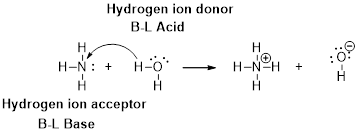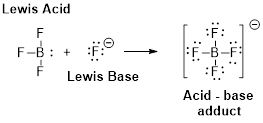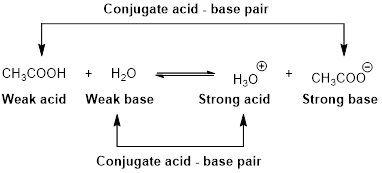# Write the formula and give the name of the conjugate base of each of the following acids. a) NH 3 b) HCO 3 − c) Br −### Chemistry & Chemical Reactivity

9th Edition
John C. Kotz + 3 others
Publisher: Cengage Learning
ISBN: 9781133949640

#### Solutions

Chapter
Section### Chemistry & Chemical Reactivity

9th Edition
John C. Kotz + 3 others
Publisher: Cengage Learning
ISBN: 9781133949640
Chapter 16, Problem 2PS
Textbook Problem
78 views

## Write the formula and give the name of the conjugate base of each of the following acids. a) NH 3 b) HCO 3 − c) Br −

(a)

Interpretation Introduction

Interpretation:

Formula and conjugated acid of given base has to be identified.

Concept Introduction:

Arrhenius Acids and Bases

Acid release hydrogen ion in water

Base release hydroxide ions in water.

HCl(aq) H+(aq) + Cl-(aq).........AcidNaOH(aq) Na+(aq)+ OH-(aq)...........Base

An acid is a substance that produces hydronium ions, H3O+ when dissolved in water.

Bronsted –Lowry definitions:

A Bronsted –Lowry acid is a proton donor, it donates a hydrogen ion, H+

A Bronsted-Lowry base is a proton acceptor, it accepts a hydrogen ion H+Lewis definition:

A Lewis acid is a substance that can accept and share an electron pair.

A Lewis base is a substance that can donate and share an electron pair.Bronsted –Lowry conjugated acid-Base pairs:

When an acid is dissolved in water, the acid (HA) donates a proton to water to form a new acid (conjugated acid) and a new base (conjugated base).The pair of an Acid –Base differs by a proton called conjugated Acid-Base pair.

### Explanation of Solution

NH3 is a base . Ammonium ion (NH4+) is a conjugated acid. NH3 and (

(b)

Interpretation Introduction

Interpretation:

Formula and conjugated acid of given base has to be identified.

Concept Introduction:

Arrhenius Acids and Bases

Acid release hydrogen ion in water

Base release hydroxide ions in water.

HCl(aq) H+(aq) + Cl-(aq).........AcidNaOH(aq) Na+(aq)+ OH-(aq)...........Base

An acid is a substance that produces hydronium ions, H3O+ when dissolved in water.

Bronsted –Lowry definitions:

A Bronsted –Lowry acid is a proton donor, it donates a hydrogen ion, H+

A Bronsted-Lowry base is a proton acceptor, it accepts a hydrogen ion H+Lewis definition:

A Lewis acid is a substance that can accept and share an electron pair.

A Lewis base is a substance that can donate and share an electron pair.Bronsted –Lowry conjugated acid-Base pairs:

When an acid is dissolved in water, the acid (HA) donates a proton to water to form a new acid (conjugated acid) and a new base (conjugated base).The pair of an Acid –Base differs by a proton called conjugated Acid-Base pair.

(c)

Interpretation Introduction

Interpretation:

Formula and conjugated acid of given base has to be identified.

Concept Introduction:

Arrhenius Acids and Bases

Acid release hydrogen ion in water

Base release hydroxide ions in water.

HCl(aq) H+(aq) + Cl-(aq).........AcidNaOH(aq) Na+(aq)+ OH-(aq)...........Base

An acid is a substance that produces hydronium ions, H3O+ when dissolved in water.

Bronsted –Lowry definitions:

A Bronsted –Lowry acid is a proton donor, it donates a hydrogen ion, H+

A Bronsted-Lowry base is a proton acceptor, it accepts a hydrogen ion H+Lewis definition:

A Lewis acid is a substance that can accept and share an electron pair.

A Lewis base is a substance that can donate and share an electron pair.Bronsted –Lowry conjugated acid-Base pairs:

When an acid is dissolved in water, the acid (HA) donates a proton to water to form a new acid (conjugated acid) and a new base (conjugated base).The pair of an Acid –Base differs by a proton called conjugated Acid-Base pair.

### Still sussing out bartleby?

Check out a sample textbook solution.

See a sample solution

#### The Solution to Your Study Problems

Bartleby provides explanations to thousands of textbook problems written by our experts, many with advanced degrees!

Get Started

Find more solutions based on key concepts
T-cells are immune cells that "read" and "remember" chemical messages to identify future invaders. T F

Nutrition: Concepts and Controversies - Standalone book (MindTap Course List)

What is a coastal cell? Where does sand in a coastal cell come from? Where does it go?

Oceanography: An Invitation To Marine Science, Loose-leaf Versin

An iron bolt of mass 65.0 g hangs from a string 35.7 cm long. The top end of the string is fixed. Without touch...

Physics for Scientists and Engineers, Technology Update (No access codes included)